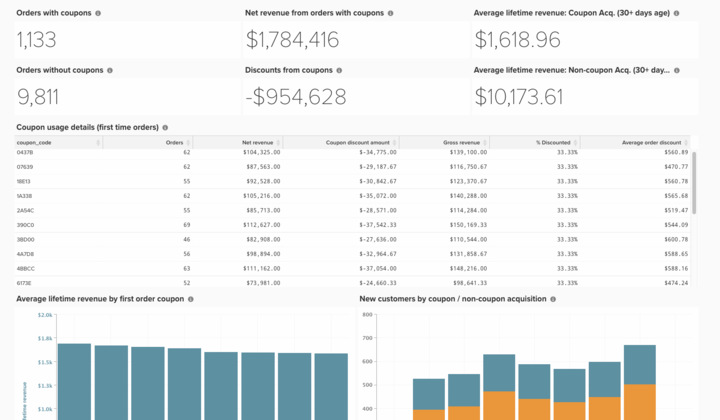# Basic Coupon Code Analysis

Understanding the coupon performance of your business is an interesting way to segment your orders and better understand customer habits.

This topic documents the steps required to create this analysis to understand how coupon-acquired customers perform, see trends, and track individual coupon code usage.## Getting Started

First, a note about how coupon codes are tracked. If a customer applied a coupon to an order, three things happen:

• A discount is reflected in the `base_grand_total` amount (your `Revenue` metric in Commerce Intelligence)
• The coupon code is stored in the `coupon_code` field. If this field is NULL (empty), the order does not have a coupon associated with it.
• The discounted amount is stored in `base_discount_amount`. Depending on your configuration, this value may appear negative or positive.

## Building a Metric

The first step is to construct a new metric with the following steps:

• Navigate to Manage Data > Metrics > Create New Metric.

• Select the `sales_order`.

• This metric performs a Sum on the base_discount_amount column, ordered by created_at.

• Filters:
• Add the `Orders we count` (Saved Filter Set)
• `coupon_code`IS NOT`[NULL]`
• Give the metric a name, such as `Coupon discount amount`.

• Once the metric has been created:

• Navigate to Dashboards > Dashboard Options > Create New Dashboard**.
• Give the dashboard a name such as `_Coupon Analysis_`.
• This is where you create and add all the reports.

## Building Reports

• New Reports:
NOTE

The Time Period** for each report is listed as `All-time`. Feel free to alter this to suit your analysis needs. Adobe recommends all reports on this dashboard cover the same time period, such as `All time`, `Year-to-date`, or `Last 365 days`.

• Orders with coupons

• Metric:`Orders`

• [`A`] `coupon_code` IS NOT `[NULL]`
• Time period: `All time`

• Interval: `None`

• Chart type:`Number (scalar)`

• Orders without coupons

• Metric: `Orders`

• [`A`] `coupon_code` IS `[NULL]`
• Time period: `All time`

• Interval:`None`

• Chart type:`Number (scalar)`

• Net revenue from orders with coupons

• Metric: `Revenue`

• [`A`] `coupon_code` IS NOT `[NULL]`
• Time period: `All time`

• Interval: `None`

• Chart type: `Number (scalar)`

• Discounts from coupons

• Metric: `Coupon discount amount`
• Time period: `All time`
• Interval: `None`
• Chart type: `Number (scalar)`
• Average lifetime revenue: Coupon acquired customers

• Metric: `Avg lifetime revenue`

• [`A`] `Customer's first order's coupon_code` IS NOT `[NULL]`
• Time period: `All time`

• Interval: `None`

• Chart type: `Number (scalar)`

• Average lifetime revenue: Non-coupon acquired customers

• Metric: `Avg lifetime revenue`

• [A] `Customer's first order's coupon_code` IS`[NULL]`
• Time period: `All time`

• Interval: `None`

• Chart type: `Number (scalar)`

• Coupon usage details (first time orders)

• Metric `1`: `Orders`

• [`A`] `coupon_code` IS NOT`[NULL]`
• [`B`] `Customer's order number` Equal to `1`
• Metric `2`: `Revenue`

• [`A`] `coupon_code` IS NOT`[NULL]`
• [`B`] `Customer's order number` Equal to `1`
• Rename: `Net revenue`

• Metric `3`: `Coupon discount amount`

• [`A`] `coupon_code` IS NOT`[NULL]`
• [`B`] `Customer's order number` Equal to `1`
• Create formula: `Gross revenue`

• Formula: `(B – C)`
• Format: `Currency`
• Create formula:% discounted

• Formula: `(C / (B - C))`
• Format: `Percentage`
• Create formula: `Average order discount`

• Formula: `(C / A)`
• Format: `Percentage`
• Time period: `All time`

• Interval: `None`

• Chart type: `Table`

• Average lifetime revenue by first order coupon

• [`A`] `coupon_code` IS`[NULL]`
• Time period: `All time`

• Interval: `None`

• Chart type: `Number (scalar)`

• Coupon usage details (first time orders)

• Metric: `Avg lifetime revenue`

• [`A`] `Customer's first order's coupon_code` IS NOT `[NULL]`
• Time period: `All time`

• Interval: `None`

• Group by: `Customer's first order's coupon_code`

• Chart type:Column

• New customers by coupon / non-coupon acquisition

• Metric `1`: `New customers`

• [`A`] `Customer's first order's coupon_code` IS NOT `[NULL]`
• Rename: `Coupon acquisition customer`

• Metric `2`: `New customers`

• [`A`] `coupon_code` IS`[NULL]`
• Rename: `Non-coupon acquisition customer`
• Time period: `All time`
• Interval: `By Month`
• Chart type: `Stacked Column`